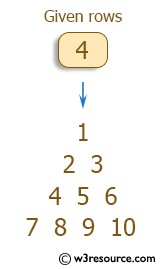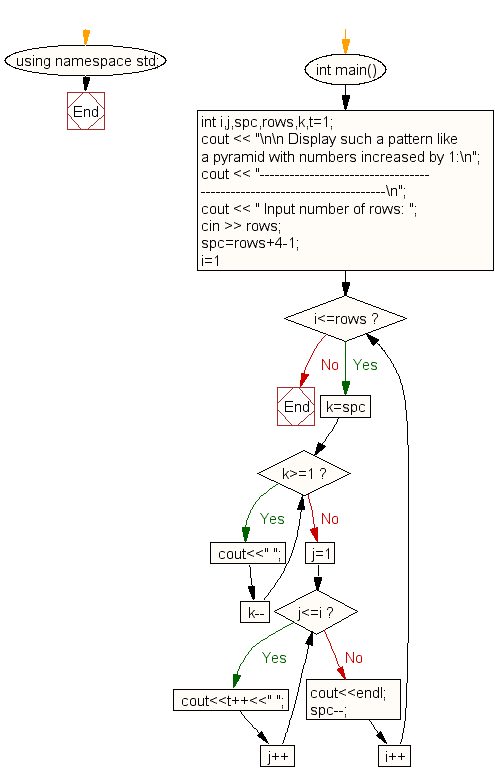﻿ C++ : Pattern like a pyramid with numbers increased by 1

# C++ Exercises: Display the pattern like a pyramid with numbers increased by 1

## C++ For Loop: Exercise-39 with Solution

Write a C++ program to make such a pattern like a pyramid with numbers increased by 1.

Pictorial Presentation:Sample Solution:

C++ Code :

``````#include <iostream>
#include <string>
using namespace std;

int main()
{
int i,j,spc,rows,k,t=1;
cout << "\n\n Display such a pattern like a pyramid with numbers increased by 1:\n";
cout << "-----------------------------------------------------------------------\n";
cout << " Input number of rows: ";
cin >> rows;
spc=rows+4-1;
for(i=1;i<=rows;i++)
{
for(k=spc;k>=1;k--)
{
cout<<" ";
}
for(j=1;j<=i;j++)
cout<<t++<<" ";
cout<<endl;
spc--;
}
}
``````

Sample Output:

``` Display such a pattern like a pyramid with numbers increased by 1:
-----------------------------------------------------------------------
Input number of rows: 4
1
2 3
4 5 6
7 8 9 10
```

Flowchart:C++ Code Editor: